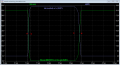Determine Instant Time on IGBT

Laz31

Joined Nov 1, 2021
1

Irving

Joined Jan 30, 2016
2,421
These are the same formulae that apply to any switching device, not just IGBTs, that go through from a high resistance to a low resistance state, or vice versa. In the course of a switching cycle the total dissipation/energy is the sum of:

$$E_{total} = E_{on} + E_{on-static} + E_{off}$$

where

$$E_{on-static} = i_c × v_{CEsat} × (t3 - t2)$$
the losses due to the on-resistance of the device.

As to the timing, this should explain it...For a first approximation the energy in the switch on or switch off phase is:

$$E_{on} = 0.5 t_{rise} × i_{on} × v_{CE-off}$$

and

$$E_{off} = 0.5 t_{fall} × i_{on} × v_{CE-off}$$

Last edited: